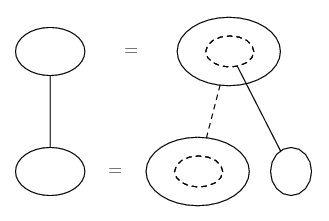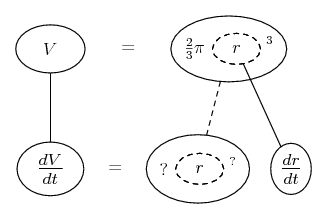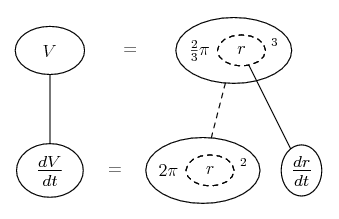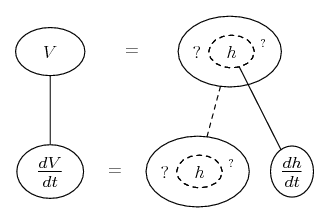# Thread: Related Rates

1. ## Related Rates

Sand falls from a hopper at a rate of 0.4 cubic meters per hour and forms a conical pile beneath. Suppose the radius of the cone is always half the height of the cone.

(a) Find the rate at which the radius of the cone increases when the radius is 4 meters.
I got (a) to be 1/(64pi)

(b) (b) Find the rate at which the height of the cone increases when the radius is 4 meters.

My roomate says I am getting this wrong but he just left.

Thanks, in advance.

2. Just in case a picture helps...

Related rates nearly always depend on the chain rule, so you might want to try filling up this pattern...... where straight continuous lines differentiate downwards (integrate up) with respect to the main variable (in this case time), and the straight dashed line similarly but with respect to the dashed balloon expression (the inner function of the composite which is subject to the chain rule).

So what have we got here? V, dV/dt, a choice of either r or h because they depend on each other, and then you're trying to find dr/dt. Writing h as 2r for part (a), the Volume formula for a cone becomes the top row here...So differentiate with respect to r...Now just replace dV/dt with 2/5 and r with 4 in the bottom row, to determine the corresponding value of dr/dt.

For part (b), get the Volume formula in terms of h, and do similarly...__________________________________________

Don't integrate - balloontegrate!

Balloon Calculus: Gallery

Balloon Calculus Drawing with LaTeX and Asymptote!

3. simply get the formula for a cone and differentiate it

diff it wrt to time. dv/dt = pi/3 (2rh dv/dt + r^2 dh/dt)
now just sub in your given values; dh/dt = 0.4 & dh/dt = 2 dr/dt (from h=2r)

you get 0.4 = pi/3 (2r^2 dr/dt + 2r^2 dr/dt)
simplifies to dr/dt = 4(0.3)/(4pir^2), now sub in r = 4

you get dr/dt = 3/160pi

for rate of height increasing, just sub back our values into the derived volume formula again since they both have r=4. i got pi/360

i havent done implicit in a wwhile so please correct me if i am wrong

#### Search Tags

rates, related